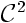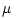## Convergence of the Continuous Time Trajectories of Isotropic Evolution Strategies on Monotonic-composite Functions

Youhei Akimoto, Anne Auger, and Nikolaus Hansen

TAO Team, INRIA Saclay-Ile-de-France, LRI, Paris Sud University, France
Youhei.Akimoto__AT__lri.fr
Anne.Auger__AT__lri.fr
Nikolaus.Hansen__AT__lri.fr

Abstract. The Information-Geometric Optimization (IGO) has been introduced as a unified framework for stochastic search algorithms. Given a parametrized family of probability distributions on the search space, the IGO turns an arbitrary optimization problem on the search space into an optimization problem on the parameter space of the probability distribution family and defines a natural gradient ascent on this space. From the natural gradients defined over the entire parameter space we obtain continuous time trajectories which are the solutions of an ordinary differential equation (ODE). Via discretization, the IGO naturally defines an iterated gradient ascent algorithm. Depending on the chosen distribution family, the IGO recovers several known algorithms such as the pure rank-update CMA-ES. Consequently, the continuous time IGO-trajectory can be viewed as an idealization of the original algorithm.

In this paper we study the continuous time trajectories of the IGO given the family of isotropic Gaussian distributions. These trajectories are a deterministic continuous time model of the underlying evolution strategy in the limit for population size to infinity and change rates to zero. On functions that are the composite of a monotone and a convex-quadratic function, we prove the global convergence of the solution of the ODE towards the global optimum. We extend this result to composites of monotone and twice continuously differentiable functions and prove local convergence towards local optima.

LNCS 7491, p. 42 ff.

© Springer-Verlag Berlin Heidelberg 2012